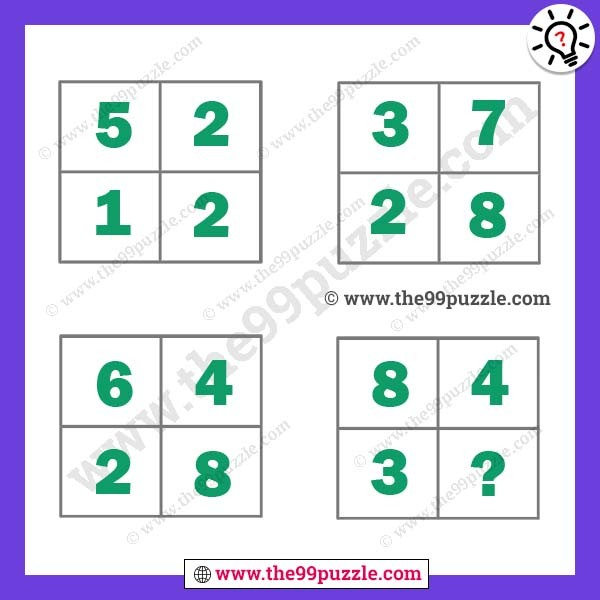# Simple logical equation math puzzle with answer – Puzz177

Simple logical equation math puzzle with the answer. Simple math logic equation can solve both kids and adults all grade students.  Tricky math riddle will challenge your brain. This math logic puzzle is dedicated to school-going students. Solving this math logic puzzle will help you to test your logical reasoning and mathematical skills. If you are intelligent people you can easily solve these puzzles?###### Explanation:

1st Box (Top Left) = 5×2+2 = 12

2nd Box (Top Right) = 3×7+7 = 28

3rd Box (Bottom Left) = 6×4+4 = 28

4th Box(Bottom Right) = 8×4+4 = 36

Here you will find many , and many more puzzles. We are creating various fun and interesting types of puzzles for adults and kids. Simple logical equation math puzzle with answer. Always think logically to solve easily these types of puzzles. On this website, you will play very fun games & quizzes that can improve your visual and logical thinking. Regularly we are updated new puzzles & quiz on this site. Check out daily to get new puzzles, riddles & quizzes.